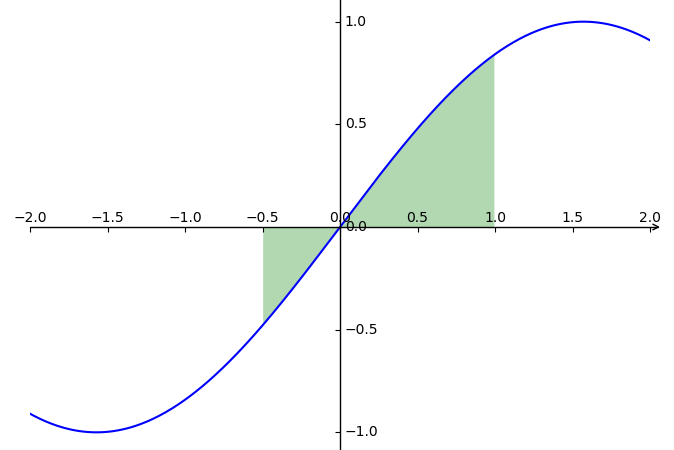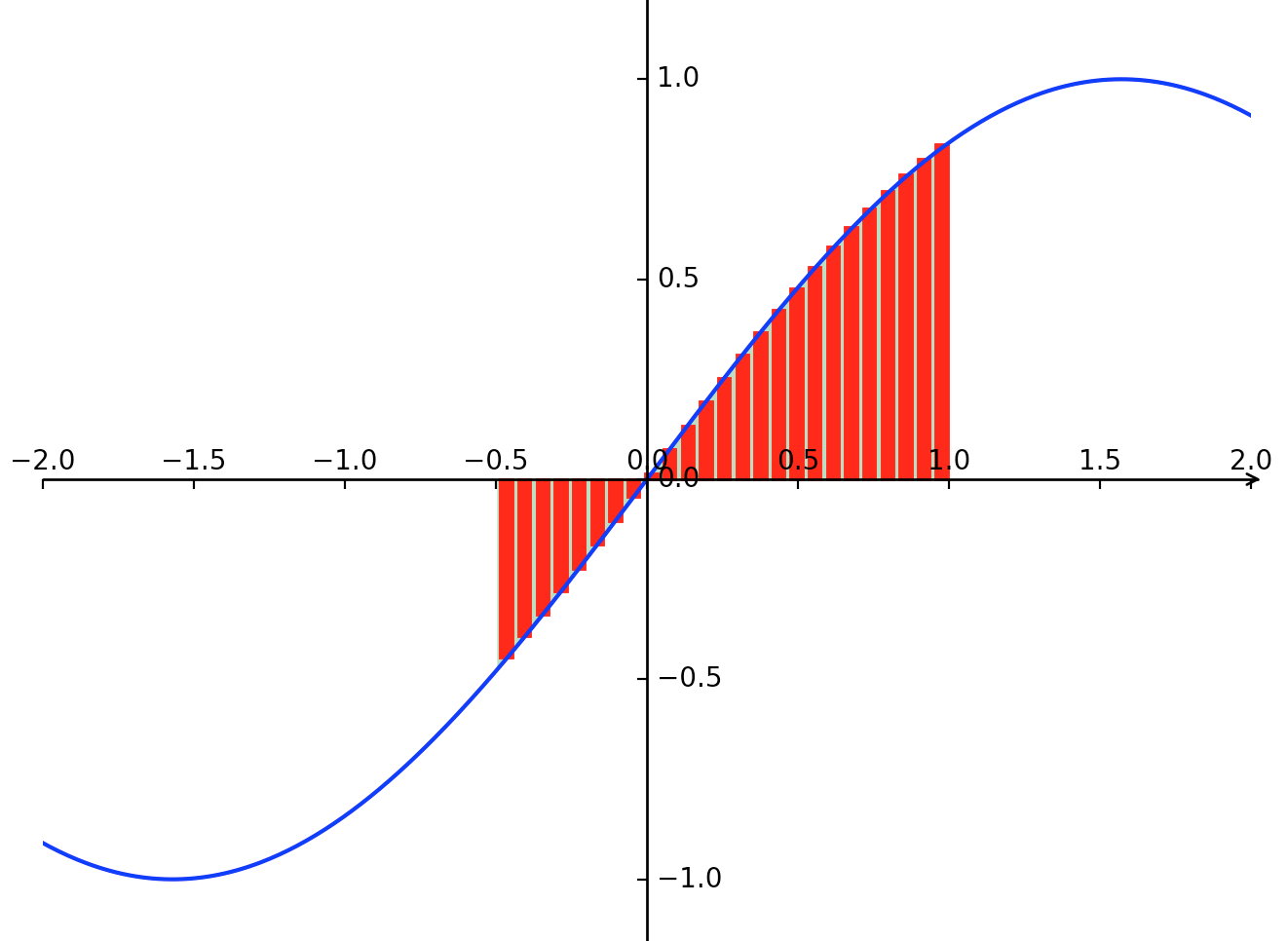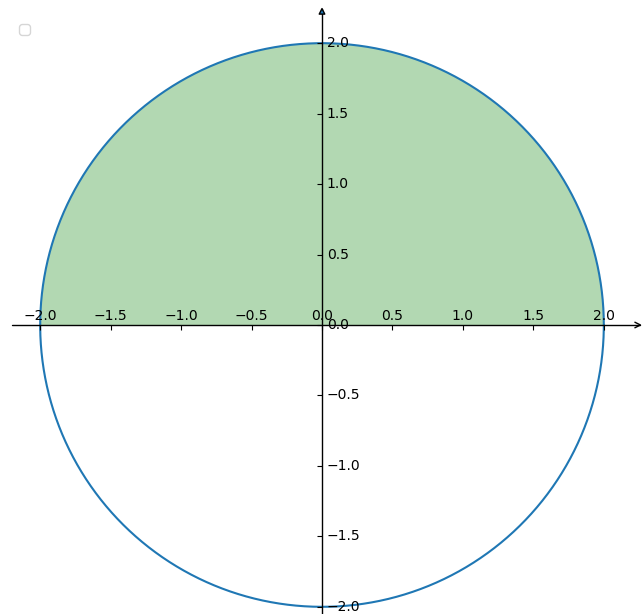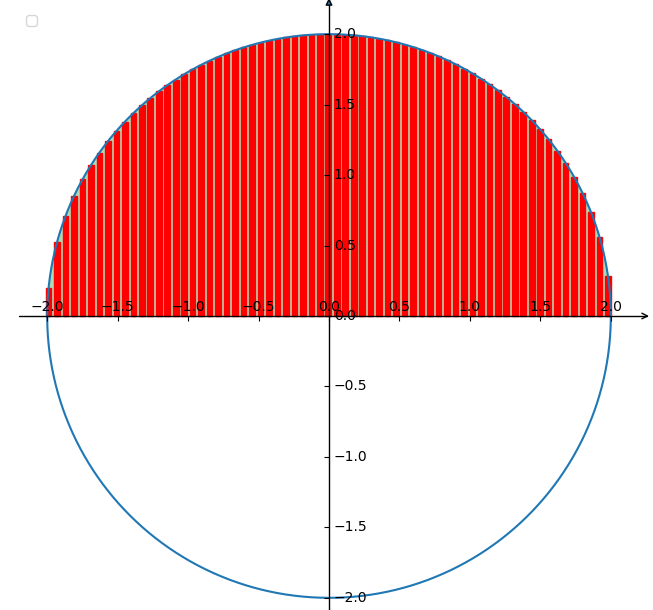# 程序实现黎曼和(定积分)#### 计算正弦曲线封闭区间和y轴相封存的面积#include <stdio.h>
#include <math.h>

// 求曲线面积(定积分)。参数为，函数f(x)，x轴上区间[begin,end]两个点的值
// @param begin    x轴区间开始位置
// @param end      x轴区间结束位置
float integration(float begin, float end)
{
float sum = 0;         //所有矩形的面积累加总和
float n = 1000;        //将函数曲线下方划为n个矩形，n值越大，精确值越高
float width = (end - begin) / n;  //单个矩形宽度，函数总长度除以矩形数量得到
for(float i=1 ; i <= n ; i++)     //计算每个矩形的面积
{
float x = begin + width * i;     //通过i的递增，得出每个矩形底部x点的值
float y = fabs(sin(x));
float area = y * width; //求x点对应的y值，取绝对值后，得到高度；再用底宽度乘高得出单个矩形面积
sum += area;         //累加矩形面积
}
return sum;
}

int main(){
printf("sin(x), x range is: [-0.5 , 1.0], area is: %f\n", integration(-0.5 , 1));
return 0;
}


$g++ test.cpp$ ./a.out
sin(x), x range is: [-0.5 , 1.0], area is: 0.582387


A = ∫ a b f ( x ) d x A = \int_a^b f(x)dx

A = ∫ a b s i n ( x ) d x A = \int_{a}^{b} sin(x)dx

A = ( − c o s ( x ) + C ) ∣ a b A = (-cos(x) + C) |_{a}^{b}

A = c o s ( a ) − c o s ( b ) A = cos(a) - cos(b)

A = ∣ c o s ( − 0.5 ) − c o s ( 0 ) ∣ + ∣ c o s ( 0 ) − c o s ( 1 ) ∣ = 0.582114 A = |cos(-0.5) - cos(0)| + |cos(0) - cos(1)| = 0.582114

#### 计算圆的面积#include <stdio.h>
#include <math.h>

// 求曲线面积(定积分)。参数为，函数f(x)，x轴上区间[begin,end]两个点的值
// @param begin    x轴区间开始位置
// @param end      x轴区间结束位置
float integration(float begin, float end)
{
float r = 2;
float a = 0;
float b = 0;
float sum = 0;         //所有矩形的面积累加总和
float n = 1000;        //将函数曲线下方划为n个矩形，n值越大，精确值越高
float width = (end - begin) / n;  //单个矩形宽度，函数总长度除以矩形数量得到
for(float i=1 ; i <= n ; i++)     //计算每个矩形的面积
{
float x = begin + width * i;     //通过i的递增，得出每个矩形底部x点的值
float y = sqrt(r*r - (x-a)*(x-a)) + b;
float area = y * width; //求x点对应的y值，取绝对值后，得到高度；再用底宽度乘高得出单个矩形面积
sum += area;         //累加矩形面积
}
return sum;
}

int main(){
printf("circle, x range is: [-2.0 , 2.0], area is: %f\n", integration(-2.0 , 2.0));
return 0;
}


circle, x range is: [-2.0 , 2.0], area is: 6.282976


# 附录

### 1. 正弦函数的图

import matplotlib.pyplot as plt
import numpy as np
import mpl_toolkits.axisartist as axisartist

#创建画布
fig = plt.figure(figsize=(8, 8))
#使用axisartist.Subplot方法创建一个绘图区对象ax
ax = axisartist.Subplot(fig, 111)
#将绘图区对象添加到画布中

# 通过set_visible方法设置绘图区所有坐标轴隐藏
ax.axis[:].set_visible(False)

# 添加x坐标轴，且加上箭头
ax.new_floating_axis
ax.axis["x"] = ax.new_floating_axis(0,0)
ax.axis["x"].set_axisline_style("->", size = 1.0)

# 添加y坐标轴，且加上箭头
ax.axis["y"] = ax.new_floating_axis(1,0)
ax.axis["y"].set_axisline_style("-|>", size = 1.0)

# 设置x、y轴上刻度显示方向
ax.axis["x"].set_axis_direction("top")
ax.axis["y"].set_axis_direction("right")

#设置x、y坐标轴的范围
plt.xlim(-2,2)
plt.ylim(-1.5,1.5)

#plt.grid() # 网格线
plt.legend(loc="upper left") # 图例说明，loc指定位置

#生成x步长为0.1的列表数据
x = np.linspace(-4, 4, 800)
y = np.sin(x)

#x - array( length N) 定义曲线的 x 坐标
#y - array( length N ) 定义曲线的 y 坐标
#如果数据点比较少的情况下，会有缝隙出现，使用interpolate可以填充缝隙
plt.fill_between(x, y, where=(-0.5<=x) & (x<=1), facecolor='green', alpha=0.3, interpolate=True)

# 绘制填充红色的矩形方块，以展示积分的直观图
qujian = x[np.where((-0.5<=x) & (x<=1))]
i = 5
for xi in qujian:
if i > 5 :
rect = plt.Rectangle((xi,0),0.04,np.sin(xi)+0.02, color='red') # 之所以给加了个+0.02，是对画出来的图微微调整，更好看些。
i = 0
i = i + 1

#绘制图形
plt.plot(x,y, c='b')

plt.show()


### 2. 圆形图

......
plt.legend(loc="upper left") # 图例说明，loc指定位置

# 圆的基本信息
# 1.圆半径
r = 2.0
# 2.圆心坐标
a, b = (0., 0.)
theta = np.arange(0, 2*np.pi, 0.01)
x = a + r * np.cos(theta)
y = b + r * np.sin(theta)
plt.plot(x, y) # 画圆
plt.axis('equal')

#x - array( length N) 定义曲线的 x 坐标
#y - array( length N ) 定义曲线的 y 坐标
#如果数据点比较少的情况下，会有缝隙出现，使用interpolate可以填充缝隙
plt.fill_between(x, y, where=(-r<=x) & (x<=r) & (y>=0), facecolor='green', alpha=0.3, interpolate=True)

# 绘制填充红色的矩形方块，以展示积分的直观图
qujian = np.linspace(-r, r, 400)
i = 5
for xi in qujian:
if i > 5 :
y = np.sqrt([(r*r - (xi-a)*(xi-a))]) + b # 根据圆的公式 r^2 = (x-a)^2 + (y-b)^2 推算出y
rect = plt.Rectangle((xi-0.02,0), 0.04, y, color='red') # 画矩形的时候有点偏差，所以往左移了0.02。
i = 0
i = i + 1

plt.show()


### 3. 除了正弦函数，我还写了余弦、指数等函数的面积计算

#include <stdio.h>
#include <math.h>

// 求曲线面积(定积分)。参数为，函数f(x)，x轴上区间[begin,end]两个点的值
// @param f(float) 这个参数是'函数指针'传值，传递的是一个函数的地址；这个函数用来求x轴上某一点对应的y值。
// @param begin    x轴区间开始位置
// @param end      x轴区间结束位置
float integration(float f(float), float begin, float end)
{
float sum = 0;         //所有矩形的面积累加总和
float n = 1000;        //将函数曲线下方划为n个矩形，n值越大，精确值越高
float width = (end - begin) / n;  //单个矩形宽度，函数总长度除以矩形数量得到
for(float i=1 ; i <= n ; i++)     //计算每个矩形的面积
{
float x = begin + width * i;     //通过i的递增，得出每个矩形底部x点的值
float area = fabs(f(x)) * width; //求x点对应的y值，取绝对值后，得到高度；再用底宽度乘高得出单个矩形面积
sum += area;         //累加矩形面积
}
return sum;
}

// 第一个例子，函数f(x)为正弦曲线
float function1(float x){
return sin(x);
}

// 第二个例子，函数f(x)为余弦曲线
float function2(float x){
return cos(x);
}

// 第三个例子，函数f(x)为指数曲线
float function3(float x){
return exp(x);
}

int main(){
printf("sin(x), x range is: [-0.5 , 1.0], area is: %f\n", integration(function1, -0.5 , 1));
printf("cos(x), x range is: [-1.0 , 1.0], area is: %f\n", integration(function2, -1 , 1));
printf("exp(x), x range is: [ 0.0 , 2.0], area is: %f\n", integration(function3, 0 , 2));
return 0;
}


$g++ test.cpp$ ./a.out
sin(x), x range is: [-0.5 , 1.0], area is: 0.582387
cos(x), x range is: [-1.0 , 1.0], area is: 1.682942
exp(x), x range is: [ 0.0 , 2.0], area is: 6.395446


• HICOOL.TOP: https://www.hicool.top/article/5dbbfdae289f2348859bee3e
• CSDN:
• 简书:
01-291616
12-29652308-24
09-03
10-315387
09-252196
10-014503
09-303205